The SURVEYREG Procedure

Variance Estimation

Subsections:

PROC SURVEYREG uses the Taylor series method or replication (resampling) methods to estimate sampling errors of estimators based on complex sample designs (Fuller 2009; Woodruff 1971; Fuller 1975; Fuller et al. 1989; Särndal, Swensson, and Wretman 1992; Wolter 2007; Rust 1985; Dippo, Fay, and Morganstein 1984; Rao and Shao 1999; Rao, Wu, and Yue 1992; Rao and Shao 1996). You can use the VARMETHOD= option to specify a variance estimation method to use. By default, the Taylor series method is used. However, replication methods have recently gained popularity for estimating variances in complex survey data analysis. One reason for this popularity is the relative simplicity of replication-based estimates, especially for nonlinear estimators; another is that modern computational capacity has made replication methods feasible for practical survey analysis.

Replication methods draw multiple replicates (also called subsamples) from a full sample according to a specific resampling scheme. The most commonly used resampling schemes are the balanced repeated replication (BRR) method and the jackknife method. For each replicate, the original weights are modified for the PSUs in the replicates to create replicate weights. The parameters of interest are estimated by using the replicate weights for each replicate. Then the variances of parameters of interest are estimated by the variability among the estimates derived from these replicates. You can use the REPWEIGHTS statement to provide your own replicate weights for variance estimation.

The following sections provide details about how the variance-covariance matrix of the estimated regression coefficients is estimated for each variance estimation method.

Taylor Series (Linearization)

The Taylor series (linearization) method is the most commonly used method to estimate the covariance matrix of the regression coefficients for complex survey data. It is the default variance estimation method used by PROC SURVEYREG.

Use the notation described in the section Notation to denote the residuals from the linear regression as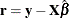with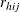as its elements. Let the
matrix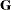be defined as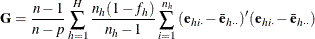where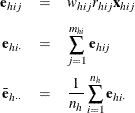The Taylor series estimate of the covariance matrix of
is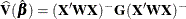The factor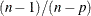in the computation of the matrixreduces the small sample bias associated with using the estimated function to calculate deviations (Hidiroglou, Fuller, and Hickman 1980). For simple random sampling, this factor contributes to the degrees of freedom correction applied to the residual mean square for ordinary least squares in which p parameters are estimated. By default, the procedure use this adjustment in the variance estimation. If you do not want to use this multiplier in variance estimation, you can specify the VADJUST=NONE option in the MODEL statement to suppress this factor.

Balanced Repeated Replication (BRR) Method

The balanced repeated replication (BRR) method requires that the full sample be drawn by using a stratified sample design with two primary sampling units (PSUs) per stratum. Let H be the total number of strata. The total number of replicates R is the smallest multiple of 4 that is greater than H. However, if you prefer a larger number of replicates, you can specify the REPS=number option. If a numbernumber Hadamard matrix cannot be constructed, the number of replicates is increased until a Hadamard matrix becomes available.

Each replicate is obtained by deleting one PSU per stratum according to the corresponding Hadamard matrix and adjusting the original weights for the remaining PSUs. The new weights are called replicate weights.

Replicates are constructed by using the first H columns of the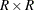Hadamard matrix . The rth (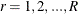) replicate is drawn from the full sample according to the rth row of the Hadamard matrix as follows:

• If the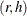element of the Hadamard matrix is 1, then the first PSU of stratum h is included in the rth replicate and the second PSU of stratum h is excluded.

• If theelement of the Hadamard matrix is –1, then the second PSU of stratum h is included in the rth replicate and the first PSU of stratum h is excluded.

Note that the "first" and "second" PSUs are determined by data order in the input data set. Thus, if you reorder the data set and perform the same analysis by using BRR method, you might get slightly different results, because the contents in each replicate sample might change.

The replicate weights of the remaining PSUs in each half-sample are then doubled to their original weights. For more details about the BRR method, see Wolter (2007) and Lohr (2010).

By default, an appropriate Hadamard matrix is generated automatically to create the replicates. You can request that the Hadamard matrix be displayed by specifying the VARMETHOD=BRR(PRINTH) method-option. If you provide a Hadamard matrix by specifying the VARMETHOD=BRR(HADAMARD=) method-option, then the replicates are generated according to the provided Hadamard matrix.

You can use the VARMETHOD=BRR(OUTWEIGHTS=) method-option to save the replicate weights into a SAS data set.

Letbe the estimated regression coefficients from the full sample for
, and let
be the estimated regression coefficient from the rth replicate by using replicate weights. PROC SURVEYREG estimates the covariance matrix ofby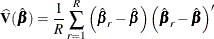with H degrees of freedom, where H is the number of strata.

Fay’s BRR Method

Fay’s method is a modification of the BRR method, and it requires a stratified sample design with two primary sampling units (PSUs) per stratum. The total number of replicates R is the smallest multiple of 4 that is greater than the total number of strata H. However, if you prefer a larger number of replicates, you can specify the REPS= method-option.

For each replicate, Fay’s method uses a Fay coefficient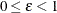to impose a perturbation of the original weights in the full sample that is gentler than using only half-samples, as in the traditional BRR method. The Fay coefficientcan be set by specifying the FAY =method-option. By default,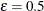if the FAY method-option is specified without providing a value for(Judkins 1990; Rao and Shao 1999). When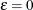, Fay’s method becomes the traditional BRR method. For more details, see Dippo, Fay, and Morganstein (1984); Fay (1984, 1989); Judkins (1990).

Let H be the number of strata. Replicates are constructed by using the first H columns of theHadamard matrix , where R is the number of replicates,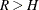. The rth () replicate is created from the full sample according to the rth row of the Hadamard matrix as follows:

• If theelement of the Hadamard matrix is 1, then the full sample weight of the first PSU in stratum h is multiplied byand the full sample weight of the second PSU is multiplied by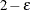to obtain the rth replicate weights.

• If theelement of the Hadamard matrix is –1, then the full sample weight of the first PSU in stratum h is multiplied byand the full sample weight of the second PSU is multiplied byto obtain the rth replicate weights.

You can use the VARMETHOD=BRR(OUTWEIGHTS=) method-option to save the replicate weights into a SAS data set.

By default, an appropriate Hadamard matrix is generated automatically to create the replicates. You can request that the Hadamard matrix be displayed by specifying the VARMETHOD=BRR(PRINTH) method-option. If you provide a Hadamard matrix by specifying the VARMETHOD=BRR(HADAMARD=) method-option, then the replicates are generated according to the provided Hadamard matrix.

Letbe the estimated regression coefficients from the full sample for. Letbe the estimated regression coefficient obtained from the rth replicate by using replicate weights. PROC SURVEYREG estimates the covariance matrix ofby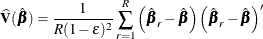with H degrees of freedom, where H is the number of strata.

Jackknife Method

The jackknife method of variance estimation deletes one PSU at a time from the full sample to create replicates. The total number of replicates R is the same as the total number of PSUs. In each replicate, the sample weights of the remaining PSUs are modified by the jackknife coefficient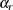. The modified weights are called replicate weights.

The jackknife coefficient and replicate weights are described as follows.

Without Stratification

If there is no stratification in the sample design (no STRATA statement), the jackknife coefficientsare the same for all replicates: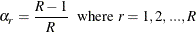Denote the original weight in the full sample for the jth member of the ith PSU as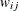. If the ith PSU is included in the rth replicate (), then the corresponding replicate weight for the jth member of the ith PSU is defined as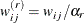With Stratification

If the sample design involves stratification, each stratum must have at least two PSUs to use the jackknife method.

Let stratum
be the stratum from which a PSU is deleted for the rth replicate. Stratumis called the donor stratum. Let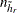be the total number of PSUs in the donor stratum
. The jackknife coefficients are defined as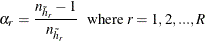Denote the original weight in the full sample for the jth member of the ith PSU as. If the ith PSU is included in the rth replicate (), then the corresponding replicate weight for the jth member of the ith PSU is defined as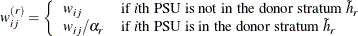You can use the VARMETHOD=JACKKNIFE(OUTJKCOEFS=) method-option to save the jackknife coefficients into a SAS data set and use the VARMETHOD=JACKKNIFE(OUTWEIGHTS=) method-option to save the replicate weights into a SAS data set.

If you provide your own replicate weights with a REPWEIGHTS statement, then you can also provide corresponding jackknife coefficients with the JKCOEFS= option. If you provide replicate weights but do not provide jackknife coefficients, PROC SURVEYREG usesas the jackknife coefficient for all replicates.

Letbe the estimated regression coefficients from the full sample for. Letbe the estimated regression coefficient obtained from the rth replicate by using replicate weights. PROC SURVEYREG estimates the covariance matrix ofby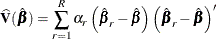with RH degrees of freedom, where R is the number of replicates and H is the number of strata, or R–1 when there is no stratification.

A Hadamard matrixis a square matrix whose elements are either 1 or –1 such that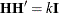where k is the dimension ofandis the identity matrix of order k. The order k is necessarily 1, 2, or a positive integer that is a multiple of 4.

For example, the following matrix is a Hadamard matrix of dimension k = 8: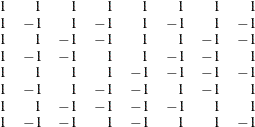Degrees of Freedom

PROC SURVEYREG produces tests for the significance of model effects, regression parameters, estimable functions specified in the ESTIMATE statement, and contrasts specified in the CONTRAST statement. It computes all these tests taking into account the sample design. The degrees of freedom for these tests differ from the degrees of freedom for the ANOVA table, which does not consider the sample design.

Denominator Degrees of Freedom

The denominator df refers to the denominator degrees of freedom for F tests and to the degrees of freedom for t tests in the analysis.

For the Taylor series method, the denominator df equals the number of clusters minus the actual number of strata. If there are no clusters, the denominator df equals the number of observations minus the actual number of strata. The actual number of strata equals the following:

• one, if there is no STRATA statement

• the number of strata in the sample, if there is a STRATA statement but the procedure does not collapse any strata

• the number of strata in the sample after collapsing, if there is a STRATA statement and the procedure collapses strata that have only one sampling unit

Alternatively, you can specify your own denominator df by using the DF= option in the MODEL statement.

For the BRR method (including Fay’s method ) without a REPWEIGHTS statement, the denominator df equals the number of strata.

For the jackknife method without a REPWEIGHTS statement, the denominator df is equal to the number of replicates minus the actual number of strata.

When there is a REPWEIGHTS statement, the denominator df equals the number of REPWEIGHTS variables, unless you specify an alternative in the DF= option in a REPWEIGHTS statement.

Numerator Degrees of Freedom

The numerator df refers to the numerator degrees of freedom for the Wald F statistic associated with an effect or with a contrast. The procedure computes the Wald F statistic for an effect as a Type III test; that is, the test has the following properties:

• The hypothesis for an effect does not involve parameters of other effects except for containing effects (which it must involve to be estimable).

• The hypotheses to be tested are invariant to the ordering of effects in the model.

See the section Testing Effects for more information. The numerator df for the Wald F statistic for a contrast is the rank of thematrix that defines the contrast.